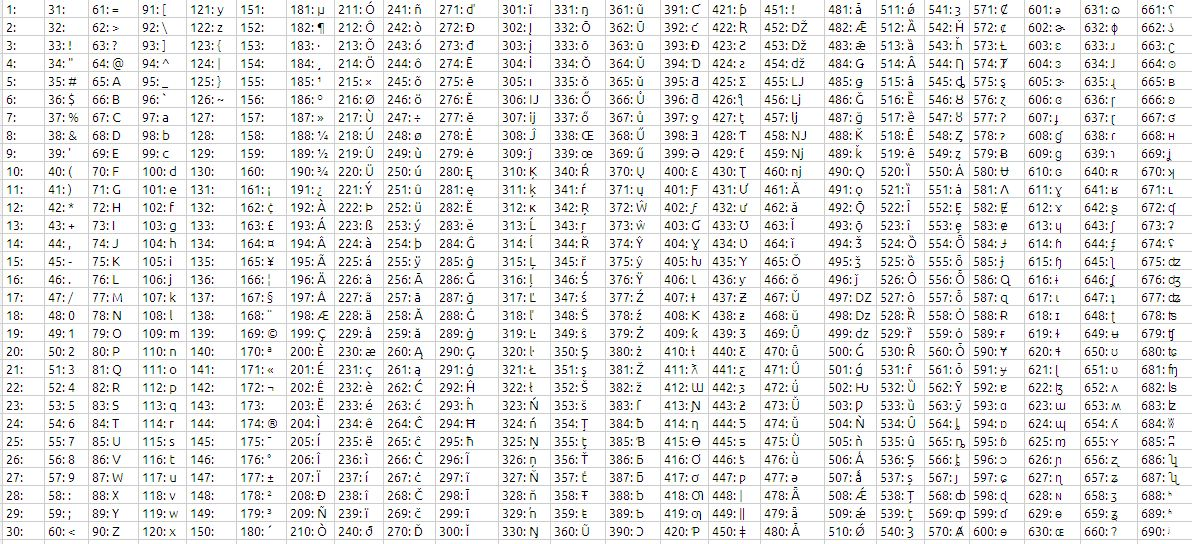# ASCII Character set in Google DocsThe formula used to generate this character set is as follows:

=ARRAYFORMULA(ROW(1:30)+(COLUMN(A:AC)*30-30)&”: “&CHAR(ROW(1:30)+(COLUMN(A:AC)*30-30)))

For each Column you will have 30 characters

the format in each cell is number followed by ASCII character